Balancing Equations Chemical Equations 5 Types of Reactions

• Slides: 34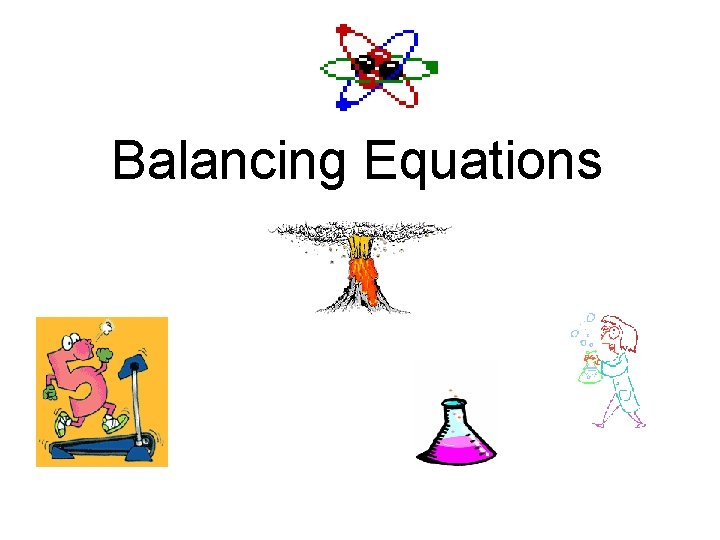Balancing Equations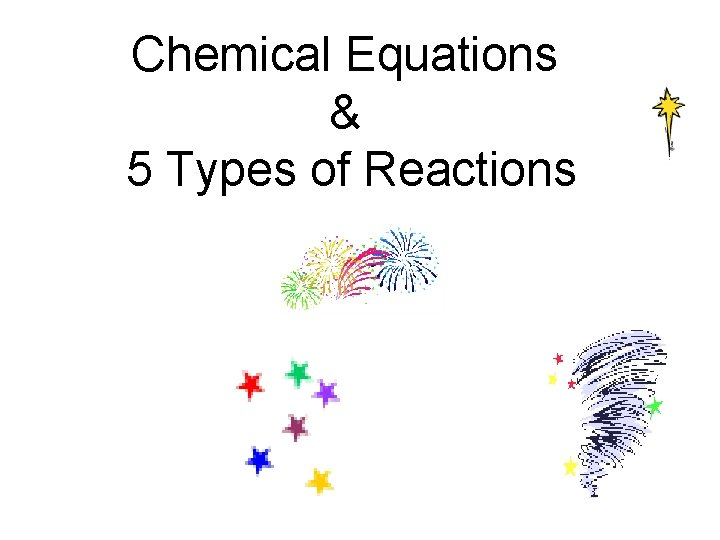Chemical Equations & 5 Types of Reactions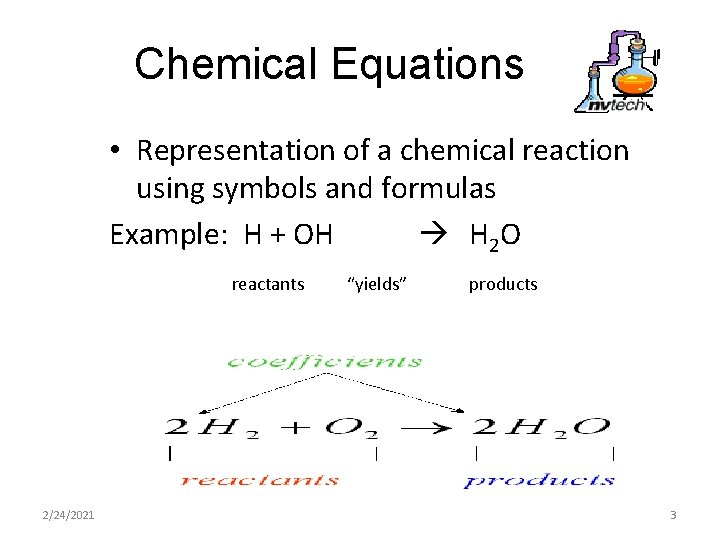Chemical Equations • Representation of a chemical reaction using symbols and formulas Example: H + OH H 2 O reactants 2/24/2021 “yields” products 3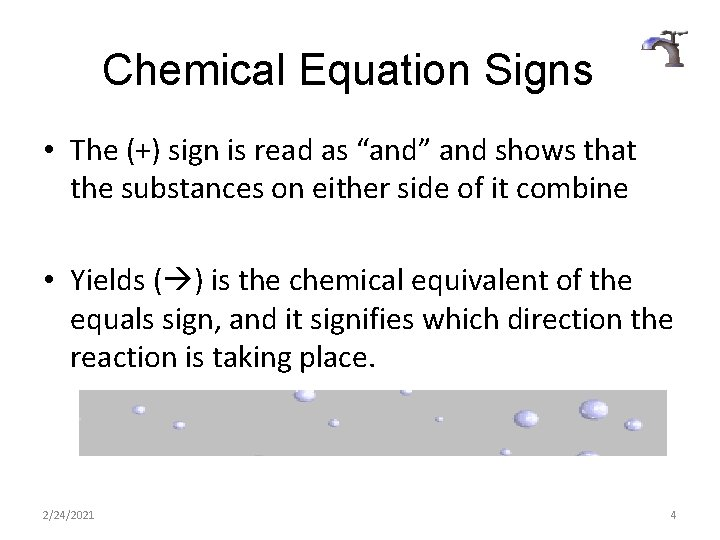Chemical Equation Signs • The (+) sign is read as “and” and shows that the substances on either side of it combine • Yields ( ) is the chemical equivalent of the equals sign, and it signifies which direction the reaction is taking place. 2/24/2021 4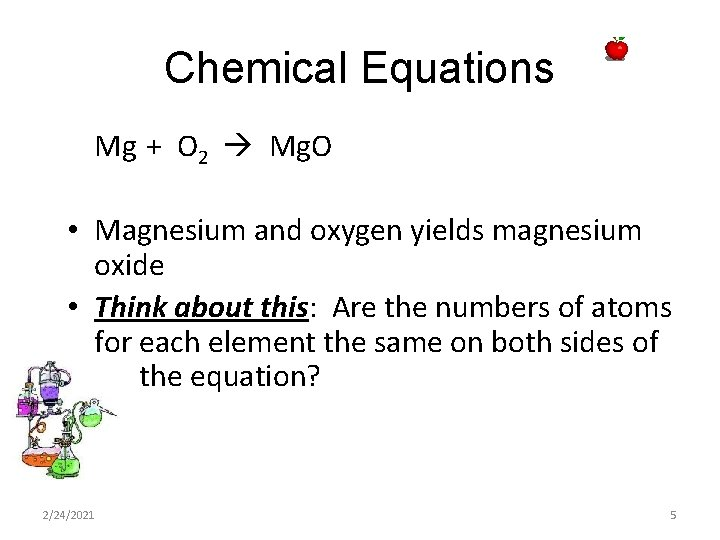Chemical Equations Mg + O 2 Mg. O • Magnesium and oxygen yields magnesium oxide • Think about this: Are the numbers of atoms for each element the same on both sides of the equation? 2/24/2021 52/24/2021 6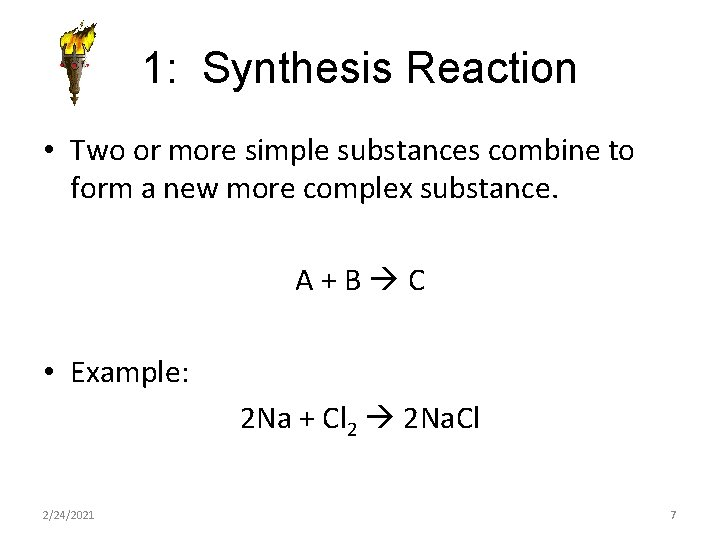1: Synthesis Reaction • Two or more simple substances combine to form a new more complex substance. A+B C • Example: 2 Na + Cl 2 2 Na. Cl 2/24/2021 7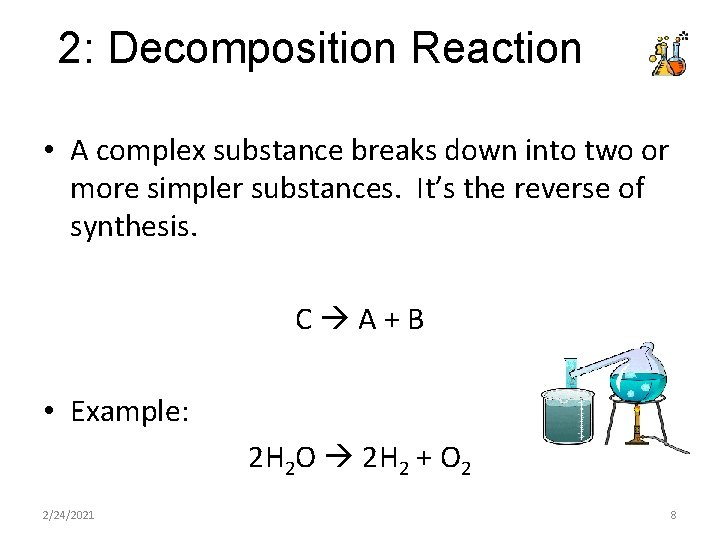2: Decomposition Reaction • A complex substance breaks down into two or more simpler substances. It’s the reverse of synthesis. C A+B • Example: 2 H 2 O 2 H 2 + O 2 2/24/2021 8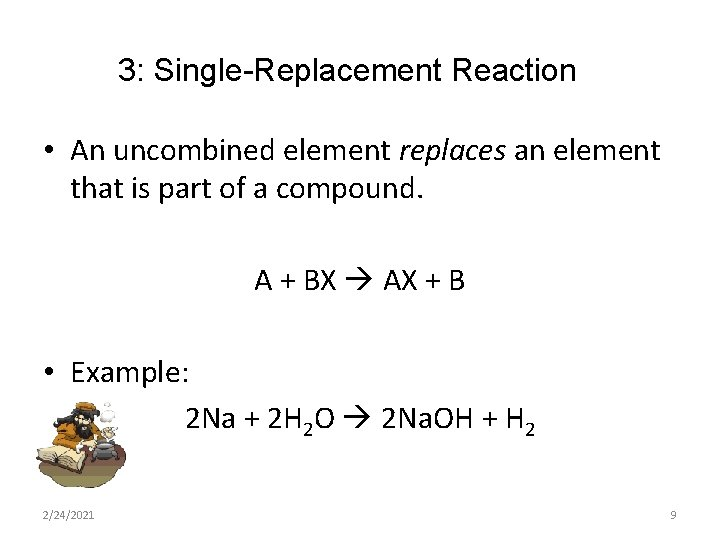3: Single-Replacement Reaction • An uncombined element replaces an element that is part of a compound. A + BX AX + B • Example: 2 Na + 2 H 2 O 2 Na. OH + H 2 2/24/2021 9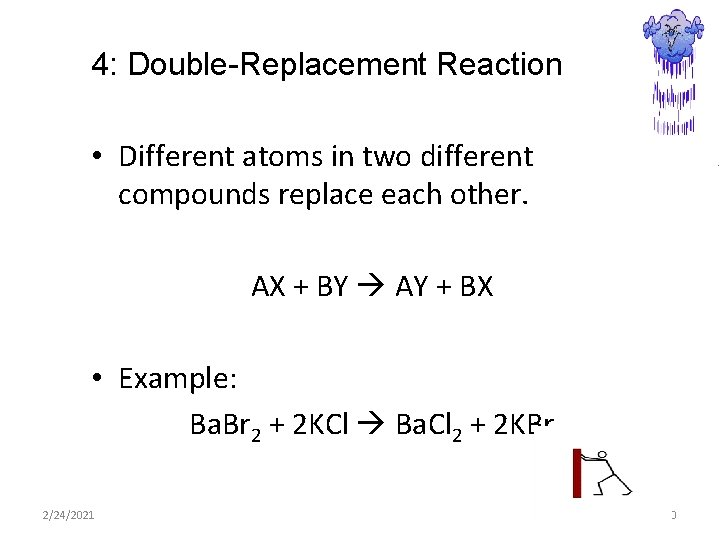4: Double-Replacement Reaction • Different atoms in two different compounds replace each other. AX + BY AY + BX • Example: Ba. Br 2 + 2 KCl Ba. Cl 2 + 2 KBr 2/24/2021 10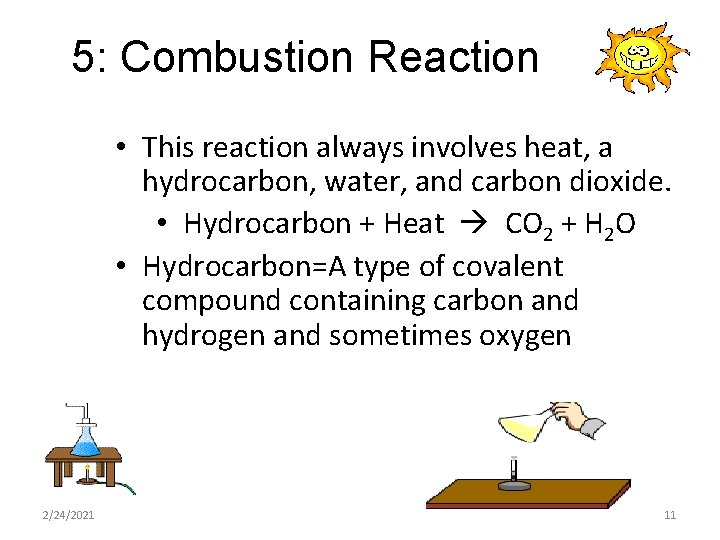5: Combustion Reaction • This reaction always involves heat, a hydrocarbon, water, and carbon dioxide. • Hydrocarbon + Heat CO 2 + H 2 O • Hydrocarbon=A type of covalent compound containing carbon and hydrogen and sometimes oxygen 2/24/2021 112/24/2021 12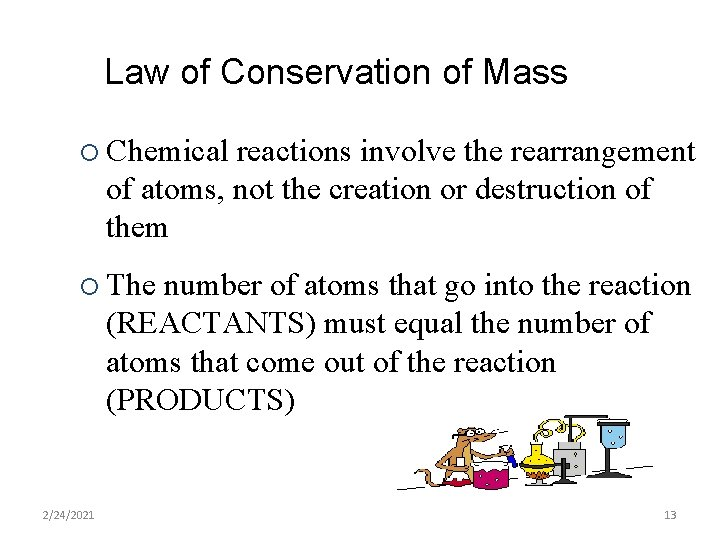Law of Conservation of Mass ¡ Chemical reactions involve the rearrangement of atoms, not the creation or destruction of them ¡ The number of atoms that go into the reaction (REACTANTS) must equal the number of atoms that come out of the reaction (PRODUCTS) 2/24/2021 13Balancing Chemical Equations Mg + O 2 Mg. O • This equation is not balanced • In order for an equation to be balanced, the number of atoms of each element must be the same on both sides of the equation. 2/24/2021 14Balancing Chemical Equations Mg + O 2 Mg. O • First count the number of atoms of each element on each side of the equation. Reactants Products Mg=1 O=2 O=1 2/24/2021 15Balancing Chemical Equations • Next, balance the number of atoms using coefficients • Coefficients are numbers placed in front of symbols and formulas • Use coefficients because changing subscripts would mean altering the substance we already have • Coefficients just change the amount of the substances we already have 2/24/2021 16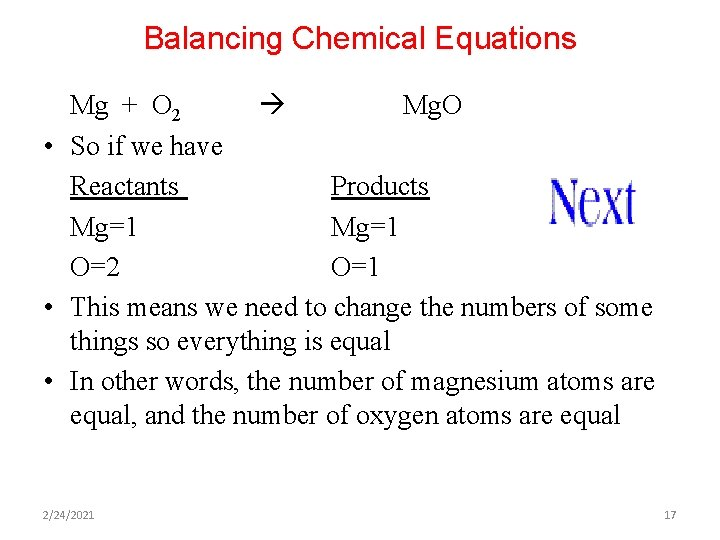Balancing Chemical Equations Mg + O 2 Mg. O • So if we have Reactants Products Mg=1 O=2 O=1 • This means we need to change the numbers of some things so everything is equal • In other words, the number of magnesium atoms are equal, and the number of oxygen atoms are equal 2/24/2021 17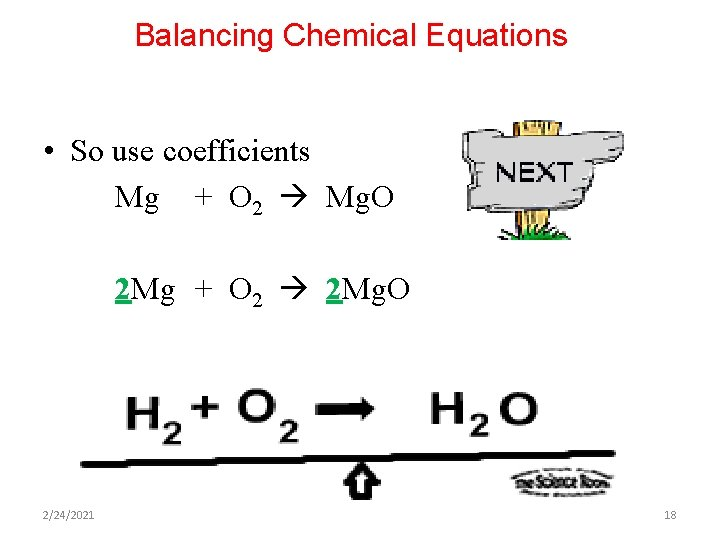Balancing Chemical Equations • So use coefficients Mg + O 2 Mg. O 2 Mg + O 2 2 Mg. O 2/24/2021 18Balancing Chemical Equations • The last thing to do, check your work by counting atoms of each element. 2 Mg + O 2 2 Mg. O Reactants Products Mg=2 O=2 • If the numbers are equal then you’re in business 2/24/2021 192/24/2021 20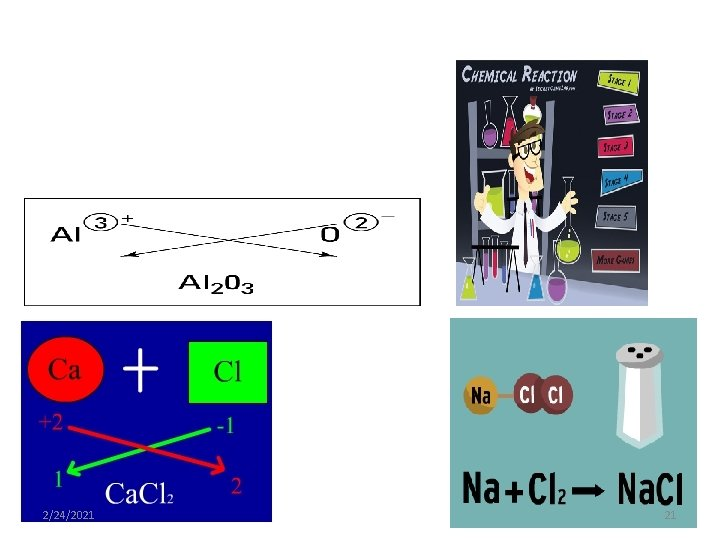2/24/2021 212/24/2021 22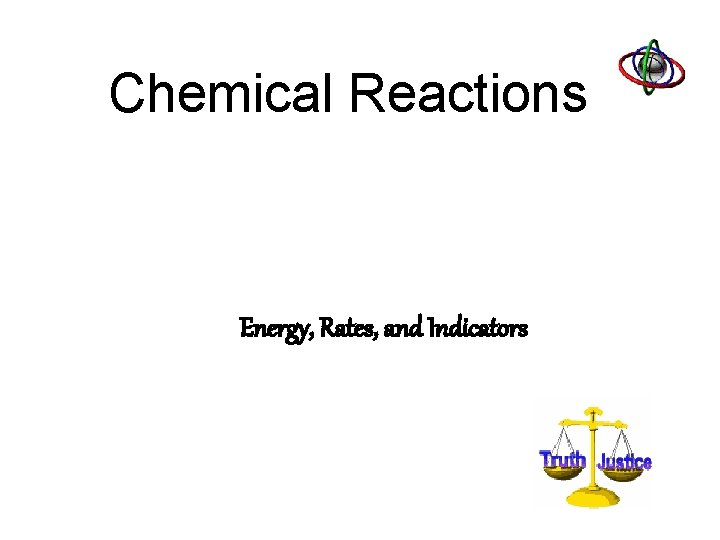Chemical Reactions Energy, Rates, and IndicatorsEnergy Conservation • Energy is always involved in a chemical reaction. Reactions either give off or absorb energy in the form of heat or light. • Law of Conservation of Energy: Either way, energy is neither created nor destroyed, it just changes form. 2/24/2021 24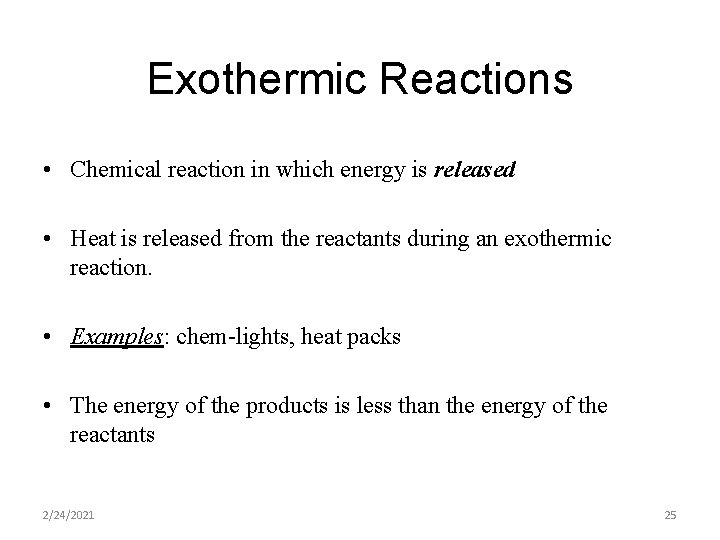Exothermic Reactions • Chemical reaction in which energy is released • Heat is released from the reactants during an exothermic reaction. • Examples: chem-lights, heat packs • The energy of the products is less than the energy of the reactants 2/24/2021 25Endothermic Reaction • Chemical reaction in which energy is absorbed • Energy is absorbed by the reactants during an endothermic reaction • Example: decomposition of sodium chloride (table salt) requires the absorption of electric energy • The energy of the products is more than the energy of the reactants 2/24/2021 26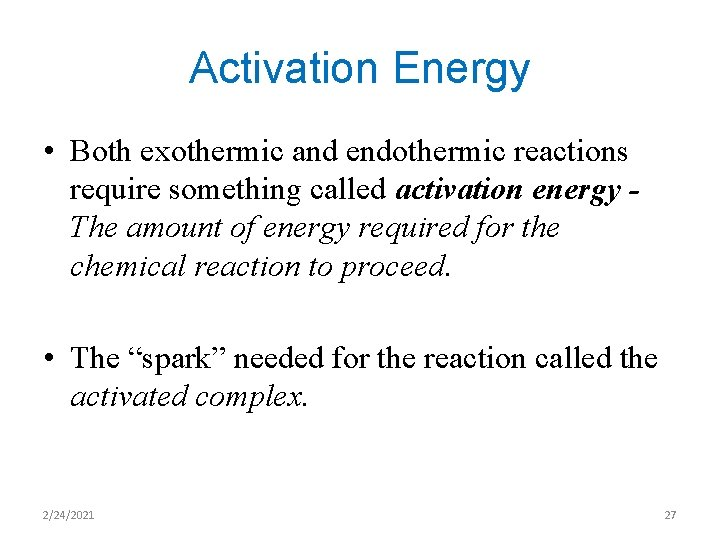Activation Energy • Both exothermic and endothermic reactions require something called activation energy The amount of energy required for the chemical reaction to proceed. • The “spark” needed for the reaction called the activated complex. 2/24/2021 27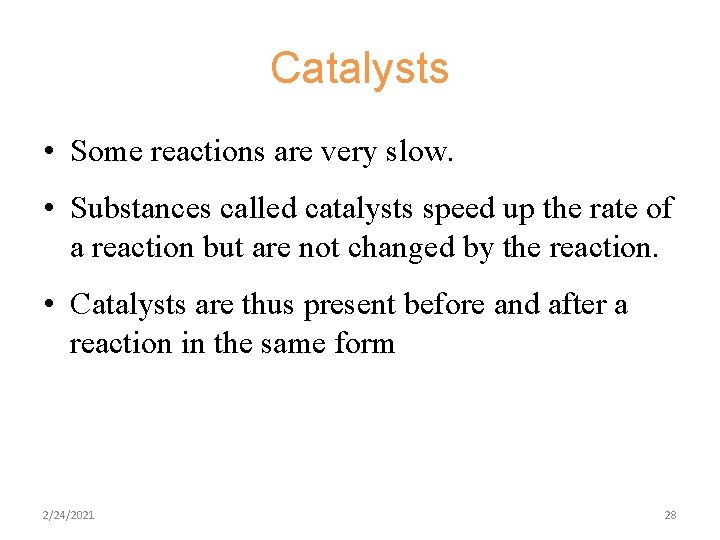Catalysts • Some reactions are very slow. • Substances called catalysts speed up the rate of a reaction but are not changed by the reaction. • Catalysts are thus present before and after a reaction in the same form 2/24/2021 28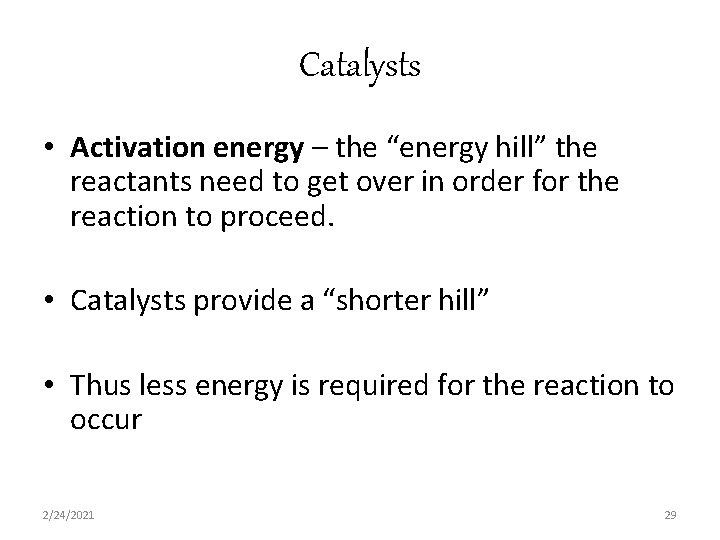Catalysts • Activation energy – the “energy hill” the reactants need to get over in order for the reaction to proceed. • Catalysts provide a “shorter hill” • Thus less energy is required for the reaction to occur 2/24/2021 29Collision Theory • Chemical reactions occur when bonds between atoms are broken, the atoms are rearranged, and new bonds are formed • In order for this to occur, atoms must collide at precisely the correct angle with the proper amount of energy • The more collisions that occur the faster the rate of the chemical reaction 2/24/2021 30Concentration and Reaction Rate • Measure of the amount of substance in a given volume • The more of a substance there is the more particles of reactants there can be for collisions • Higher concentrations of reactants mean higher reaction rates 2/24/2021 31Surface Area and Reaction Rate • Which dissolves faster? sugar cube grounded sugar • Increases in surface area means a faster reaction rate • Medicines work this way 2/24/2021 32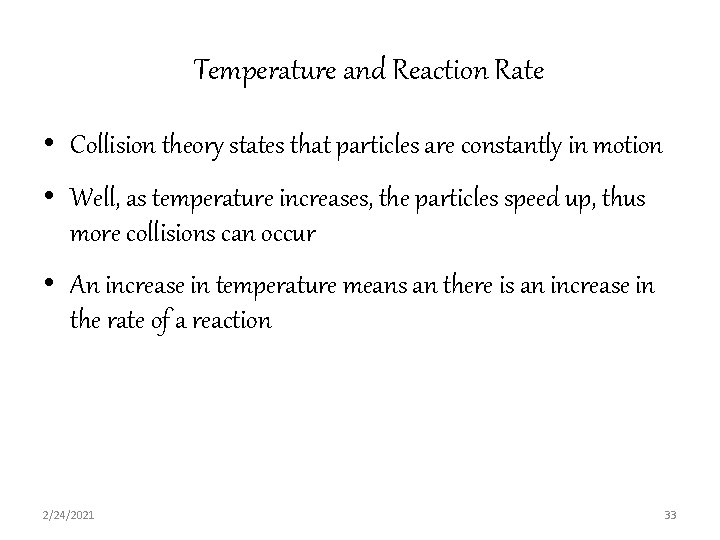Temperature and Reaction Rate • Collision theory states that particles are constantly in motion • Well, as temperature increases, the particles speed up, thus more collisions can occur • An increase in temperature means an there is an increase in the rate of a reaction 2/24/2021 33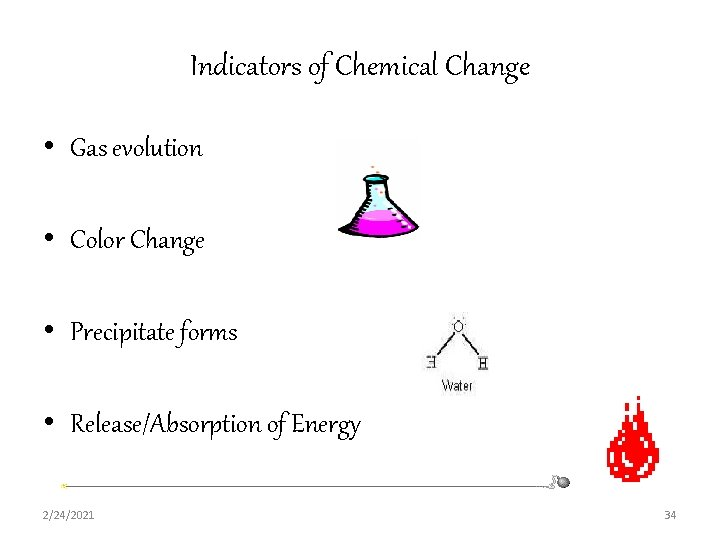Indicators of Chemical Change • Gas evolution • Color Change • Precipitate forms • Release/Absorption of Energy 2/24/2021 34